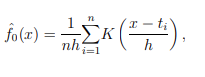# RooKeyPdf with a parameter shift

Dear RooFit Experts,
I am trying to create a RooKeyPdf as a function of a
RooFormulaVar( x + shift)
where x has been used to construct the dataset and the shift is centered at 0, and let being floating later on in the main fit.
In practice i am looking up to apply a shift on the RooKeyPdf i create so that the fit will translate lef/right the rookey to find the best fitting point.
I have an issue , when creating

``````auto *shiftedX =  new RooFormulaVar(TString::Format("shifted_mass_%s", _parsedShiftKey.Data()), "@0+@1", RooArgList(*m_var, *shiftPar )));
m_pdf = new RooKeysPdf(_pdfName, _pdfName, *shiftedX, *m_data, _mirrorOption, _rho);
``````

I tried also using RooAddition to create the RooKeyPdf but again the code segfault.
Is there a way out ?
Can one take the implementation of the RooKeyPdf and do minimal changes to the intenral code to have a
RooShiftableKeyPdf function ?
Thanks
Renato

More specifically, using a RooKeyPdf with a RooFormulaVar, it seffault,
It throws

``````terminate called after throwing an instance of 'std::length_error'
what():  vector::reserve
Annullato (core dumped)
``````

maybe, just guessing i need to use

`````` RooKeysPdf::RooKeysPdf(const char *name, const char *title,
RooAbsReal& xpdf, RooRealVar& xdata, RooDataSet& data,
Mirror mirror, Double_t rho) :
``````

this constructor? Where xpdf is a possibel transformation of the var and xdata is the actual x over which the RooKey is computed?
The second constructor seems to work, and the fit runs up to the end, however i wonder if it’s preferable to use RooAddition or RooFormulaVar.

I come back to this, i see a strange behaviour i don’t understand :
Attached macro to see what i mean :
test_shiftRooKey.C (2.1 KB)

It seems like the constructor is reverting the order of parameters.
Can someone help me to understand how to best achieve this?
In the macro :

• generate a gaussian with centered at 0 mean value,
• create a RooKeyPdf passing the shifted option setup (rookey initially centered at 0 )
• generate data with shifted mean at 3
• Fit the RooKey to the shifted dataset,
The issue i have , which is a bit counterintuitive is about hte sign the shift has when fitting:
i.e with
``````   RooFormulaVar shiftMean( "shiftMean", "@0-@1", RooArgList(x, shiftPar));
``````

the shiftPar converge to +3.
while
i.e with

``````   RooFormulaVar shiftMean( "shiftMean", "@0+@1", RooArgList(x, shiftPar));
``````

It converge to -3. It’s a bit counterintuitive, since i would expect the `@0+@1` setup to give me the +3 as fitted shift value.
I ping @moneta and @jonas as you might have an idea if i am doing something wrong here.

I modified my script, I think that RooKeyPdf and Gaussian with a “mean+shift” has 2 different meanings, and if I want to share the “mass shifts” among 2 fitting PDFs , the RooKeyPdf has to be constricted with a reverted sign on the shift :
I,E

``````   RooRealVar shiftPar("shift", "shift", 0, -20, 20);
RooFormulaVar shiftMean( "shiftMean", "@0+@1", RooArgList(x,shiftPar )); //HERE
RooFormulaVar shiftParFit( "shiftPar", "@0+@1", RooArgList(mean,shiftPar )); //HERE
RooGaussian gauss_allowmeanshift("gauss", "gaussian PDF", x, shiftParFit, sigma);
``````

If i fit the shifted data with gauss_allowmeanshift, i get shift = +3
If i fit the shifted data with rookeypdf constructed with + sign, i get shift = -3
I guess this is an issue concerning “relative” to what you are shifting, so for fitting parameters of a pdf it has a sign, while if the shift is wrt the “observable” of the fit itself, it has to revert the sign.

Hi,
I am not sure why you would need to shift the keys pdf.
I think it makes sense te sign is reversed because the density is estimated viaIf you shift `x` is equivalent to shift the data `t` by the opposite sign, which is what you effectivly are doing. So your results make sense to me.

Lorenzo

Thanks a lot @moneta , indeed i have a final PDF with
Model(Analytical) + SomeBackground(RooKeyPdfs) and a m_shift introduced with `mean + m_shift` on the Model to parameterise the mass shift due to detector effects.
I want the shift it converges to to be shared on the RooKeyPdfs i construct for the backgrounds so that any signal translation , is also a background translation.
Therefore, i think that this can be accomplished having a

``````mean + mshift
``````

for analytical PDFs, AND constructing the RooKeyPdf with the `x - m_shift` instead
This would align the shift sign , correct?

PS :
thanks a lot for the feedback

Hi,

Yes it is correct. I understand now your use case. Theoretically the correct thing will be to shift the data, input to the RooKeysPdf. This is equivalent to an opposite shift on the input x parameter.

Lorenzo

1 Like

The only difference i guess is that when minimizing the Likelihood for a shift on the parameter, it will keep translating the rookeypdf to find the proper minimum. If you create the RooKey to model data with a shifted input observable already, you need to know in advance the shift at which the fit would converge before actually running the fit. That’s why i have this use case

Hi,
I fully understand your use case. RooFit does not support the capability to parametrize the input data of the RooKeyPdf to modify them at every minimization iteration, so you need to parametrize the input.

1 Like

This topic was automatically closed 14 days after the last reply. New replies are no longer allowed.